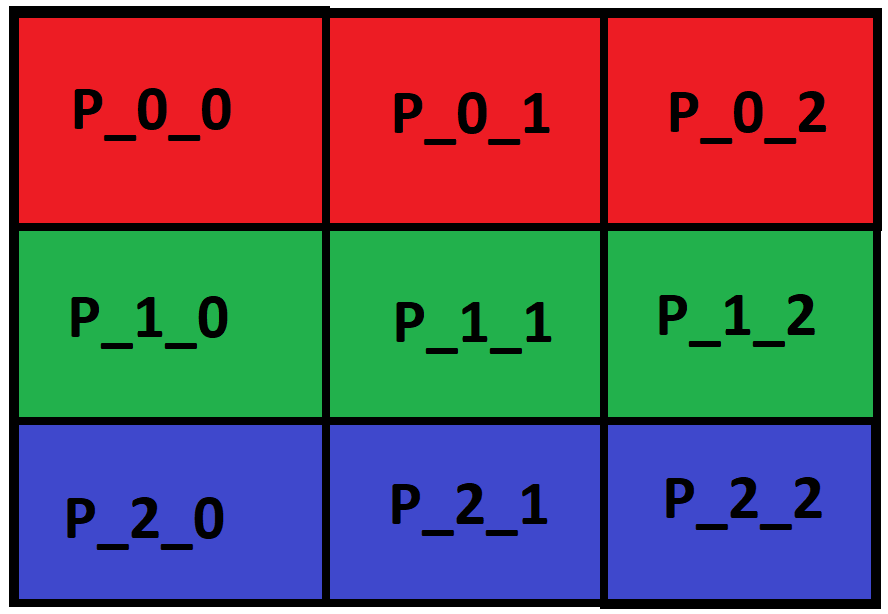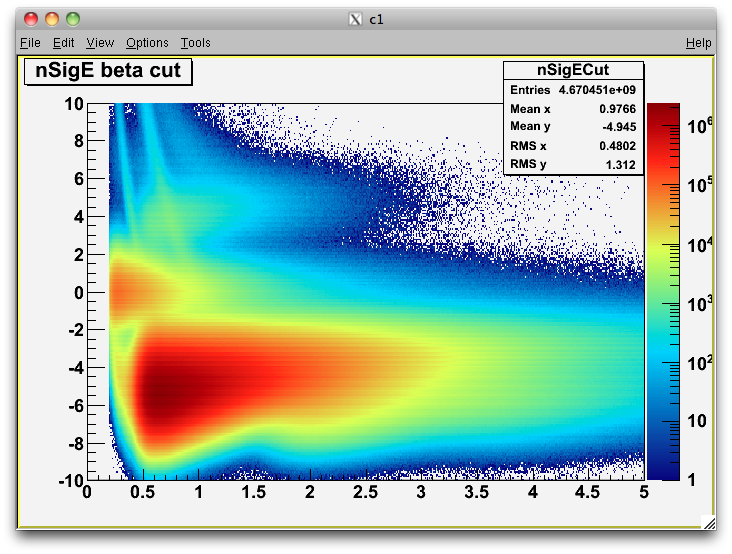# QPainter: Colored matrix to paint

• I'm learning about how to paint a widget using `QPainter` (pixel per pixel) and I already saw the `Basic Tools Plugin` example in the Qt Creator, but I'm a little bit confuse about how to do this simple task below:

``````std::vector< std::vector<QColor> > colored_matrix(3, 3);

// Fill the 'colored_matrix' with QColor data (RGB)
// Red:
colored_matrix = QColor(256, 0, 0, 0);
colored_matrix = QColor(256, 0, 0, 0);
colored_matrix = QColor(256, 0, 0, 0);
// Green:
colored_matrix = QColor(0, 256, 0, 0);
colored_matrix = QColor(0, 256, 0, 0);
colored_matrix = QColor(0, 256, 0, 0);
// Blue:
colored_matrix = QColor(0, 0, 256, 0);
colored_matrix = QColor(0, 0, 256, 0);
colored_matrix = QColor(0, 0, 256, 0);

// Paint this matrix in a UI widget.
``````How can I get this output colored matrix UI (just the colors, without the cells names)?

• @fem_dev here is the solution!

``````    // Set the image size:
int sizeX = 400;
int sizeY = 400;

// Create and init the image:
QImage image = QImage(sizeX, sizeY, QImage::Format_RGB32);

// Fill the image with colors:
for (int x = 0; x < sizeX; x++) {
for (int y = 0; y < sizeY; y++) {
image.setPixel(x, y, qRgb(x, y, 200));
}
}

// Create a graphics scene:
QGraphicsScene* graphic = new QGraphicsScene(this);

// Set the image:

// Display it on the UI:
ui->graphicsView->setScene(graphic);
``````

• Hi,

Looks like you need two for loops that will draw the rectangles and pick the color from your matrix.

Do you need any more details ?

• @SGaist yes...I understand the generic algorithm, but I don't know how to paint it on UI screen.

Could you help me?

• @fem_dev here is the solution!

``````    // Set the image size:
int sizeX = 400;
int sizeY = 400;

// Create and init the image:
QImage image = QImage(sizeX, sizeY, QImage::Format_RGB32);

// Fill the image with colors:
for (int x = 0; x < sizeX; x++) {
for (int y = 0; y < sizeY; y++) {
image.setPixel(x, y, qRgb(x, y, 200));
}
}

// Create a graphics scene:
QGraphicsScene* graphic = new QGraphicsScene(this);

// Set the image:

// Display it on the UI:
ui->graphicsView->setScene(graphic);
``````

• You are over-complicating things.

If you want to use a QGraphicsScene, then use QGraphicsScene::addRect and pass the colour to the brush.

Otherwise, QImage provides a fill method.

For QPainter, you have QPainter::drawRect. Just modify the painter brush before paiting the rectangle.

• @SGaist thank you. For my given colored matrix above you are right!

I called this post above as a "solution" because, in the original first post, I was talking about a oversimplified view of my real problem. It was my fault!
I tried to simplify the real problem a lot and I ended up changing the focus / resolution target. Sorry about that!

Well, I want to develop a 2D histogram graph using Qt. So I have to set a color pixel per pixel on screen, like this example below:Using the source-code in this post above I can set the color values pixel per pixel.

QUESTION:
Is there a better/correct way to do this?

In the nexts steps I need to understand how to:
a) Create the X and Y axis (with ticks ans labels)
b) Create the right-side color pallet (with ticks ans labels)
c) Set a graph title

Please, if you have any sugestions about how to implement these things, let me know.

Thank you again!

• It's always nice to see ROOT plots ;-)

It open the Windows Console and give a error about `missing #include <new>` and another things....and closes the console window...